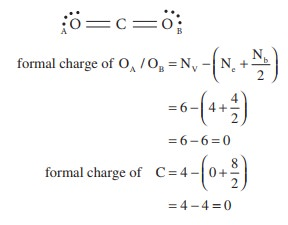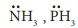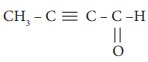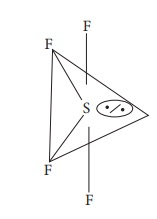# UNIT 10: Chemical bonding - Online Test

Q1. In which of the following Compounds does the central atom obey the octet rule?
Explaination / Solution:

Compound  : No. of valance electron on the central atom

XeF2 : 10

AlCl3 : 6

SF6 : 12

SCl2 : 8

Q2. In the molecule OA == C==OB, the formal charge on OA, C and OB are respectively.
Explaination / Solution:Q3. Which of the following is electron deficient?
Explaination / Solution:- electron rich,

CH3 – CH3 – Covalent neutral molecule.

BH3 - electron deficient

Q4. Which of the following molecule contain no л bond?
Explaination / Solution:water contain only σ bonds and no π bonds option

Q5. The ratio of number of sigma (σ) and pi (л) bonds in 2- butynal is
Explaination / Solution:2-butynal

no. of σ bonds = 8[4C-H;,3C-C;1C-O]

no. of π bonds = 3[2C-C; 1C-O]

ratio=8/3

Q6. Which one of the following is the likely bond angles of sulphur tetrafluoride molecule?
Explaination / Solution:Normal bond angle in regular trigonal bipyramidal are 90º and 120 ºdue to l.p - b.p repulsion, bond angle reduced 89 º, 117 º

Q7.

Assertion: Oxygen molecule is paramagnetic.

Reason : It has two unpaired electron in its bonding molecular orbital

Explaination / Solution:

Correct Statement :

Oxygen molecule is paramagnetic Correct Reason

It has two unpaired electron in its antibond-ing molecular orbital.

Option (c) assertion true and reason false.

Q8. According to Valence bond theory, a bond between two atoms is formed when
Explaination / Solution:

two half filled orbitals overlap.

Q9. In ClF3 ,NF3 and BF3 molecules the chlorine, nitrogen and boron atoms are
Explaination / Solution:

ClF3 – Sp3d hybridisation

NF3 - Sp3 hybridisation

BF3 - Sp2 hybridisation

Q10. When one s and three p orbitals hybridise,
Explaination / Solution:

Option (b) is correct Sp3 hybridisation.

Oribital geometry tetrahedron, bond angle 109º28'